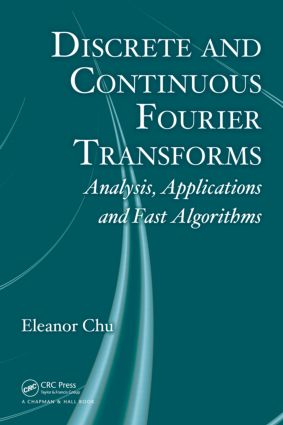Discrete and Continuous Fourier Transforms

Analysis, Applications and Fast Algorithms, 1st Edition

Chapman and Hall/CRC

424 pages | 115 B/W Illus.

Hardback: 9781420063639
pub: 2008-03-19
Currently out of stock
\$135.00
x
eBook (VitalSource) : 9780429144752
pub: 2008-03-19
from \$28.98

FREE Standard Shipping!

Description

Long employed in electrical engineering, the discrete Fourier transform (DFT) is now applied in a range of fields through the use of digital computers and fast Fourier transform (FFT) algorithms. But to correctly interpret DFT results, it is essential to understand the core and tools of Fourier analysis. Discrete and Continuous Fourier Transforms: Analysis, Applications and Fast Algorithms presents the fundamentals of Fourier analysis and their deployment in signal processing using DFT and FFT algorithms.

This accessible, self-contained book provides meaningful interpretations of essential formulas in the context of applications, building a solid foundation for the application of Fourier analysis in the many diverging and continuously evolving areas in digital signal processing enterprises. It comprehensively covers the DFT of windowed sequences, various discrete convolution algorithms and their applications in digital filtering and filters, and many FFT algorithms unified under the frameworks of mixed-radix FFTs and prime factor FFTs. A large number of graphical illustrations and worked examples help explain the concepts and relationships from the very beginning of the text.

Requiring no prior knowledge of Fourier analysis or signal processing, this book supplies the basis for using FFT algorithms to compute the DFT in a variety of application areas.

Preface

Fundamentals, Analysis, and Applications

Analytical and Graphical Representation of Function Contents

Time and Frequency Contents of a Function

The Frequency-Domain Plots as Graphical Tools

Identifying the Cosine and Sine Modes

Using Complex Exponential Modes

Using Cosine Modes with Phase or Time Shifts

Periodicity and Commensurate Frequencies

Review of Results and Techniques

Expressing Single Component Signals

General Form of a Sinusoid in Signal Application

Fourier Series: A Topic to Come

Terminology

Sampling and Reconstruction of Functions—Part I

DFT and Band-Limited Periodic Signal

Frequencies Aliased by Sampling

Connection: Anti-Aliasing Filter

Alternate Notations and Formulas

Sampling Period and Alternate Forms of DFT

Sample Size and Alternate Forms of DFT

The Fourier Series

Formal Expansions

Time-Limited Functions

Even and Odd Functions

Half-Range Expansions

Fourier Series Using Complex Exponential Modes

Complex-Valued Functions

Fourier Series in Other Variables

Truncated Fourier Series and Least Squares

Orthogonal Projections and Fourier Series

Convergence of the Fourier Series

Accounting for Aliased Frequencies in DFT

DFT and Sampled Signals

Deriving the DFT and IDFT Formulas

Direct Conversion between Alternate Forms

DFT of Concatenated Sample Sequences

DFT Coefficients of a Commensurate Sum

Frequency Distortion by Leakage

Computing DFT Defining Formulas Per Se

Sampling and Reconstruction of Functions—Part II

Sampling Nonperiodic Band-Limited Functions

Deriving the Fourier Transform Pair

The Sine and Cosine Frequency Contents

Tabulating Two Sets of Fundamental Formulas

Connections with Time/Frequency Restrictions

Fourier Transform Properties

Alternate Form of the Fourier Transform

Computing the Fourier Transform

Computing the Fourier Coefficients

Sampling and Reconstruction of Functions—Part III

Impulse Functions and Their Properties

Generating the Fourier Transform Pairs

Convolution and Fourier Transform

Periodic Convolution and Fourier Series

Convolution with the Impulse Function

Impulse Train as a Generalized Function

Impulse Sampling of Continuous-Time Signals

Nyquist Sampling Rate Rediscovered

Sampling Theorem for Band-Limited Signal

Sampling of Band-Pass Signals

The Fourier Transform of a Sequence

Deriving the Fourier Transform of a Sequence

Properties of the Fourier Transform of a Sequence

Generating the Fourier Transform Pairs

Duality in Connection with the Fourier Series

The Fourier Transform of a Periodic Sequence

The DFT Interpretation

The Discrete Fourier Transform of a Windowed Sequence

A Rectangular Window of Infinite Width

A Rectangular Window of Appropriate Finite Width

Frequency Distortion by Improper Truncation

Windowing a General Nonperiodic Sequence

Frequency-Domain Properties of Windows

Applications of the Windowed DFT

Discrete Convolution and the DFT

Linear Discrete Convolution

Periodic Discrete Convolution

The Chirp Fourier Transform

Applications of the DFT in Digital Filtering and Filters

The Background

Application-Oriented Terminology

Revisit Gibbs Phenomenon from the Filtering Viewpoint

Experimenting with Digital Filtering and Filter Design

Fast Algorithms

Algebraic DFT versus FFT-Computed DFT

The Role of Index Mapping

The Recursive Equation Approach

Other Forms by Alternate Index Splitting

Kronecker Product Factorization and FFTs

From Two-Factor to Multifactor Mixed-Radix FFT

Other Forms by Alternate Index Splitting

Factorization Results by Alternate Expansion

Unordered FFT for Scrambled Input

Utilities of the Kronecker Product Factorization

The Family of Prime Factor FFT Algorithms

Connecting the Relevant Ideas

Deriving the Two-Factor PFA

Matrix Formulation of the Two-Factor PFA

Matrix Formulation of the Multifactor PFA

Number Theory and Index Mapping by Permutations

The In-Place and In-Order PFA

Efficient Implementation of the PFA

On Computing the DFT of Large Prime Length

Performance of FFT for Prime N

Fast Algorithm I: Approximating the FFT

Fast Algorithm II: Using Bluestein’s FFT

Bibliography

Index